# MOTION 1 Motion and Rest 2 Distance and

• Slides: 39
Download presentationMOTION 1. Motion and Rest 2. Distance and Displacement 3. Uniform Motion 4. Non-uniform Motion 5. Speed 6. Velocity 7. Acceleration 8. Equations of Uniformly Accelerated Motion 9. Graphical Representation of Motion 10. Distance-Time Graph 11. Speed-Time Graph 12. Derivation of Equations of Motion by Graphical Method 13. Uniform Circular Motion 14. Calculation of Speed of a Body in Uniform Circular Motion Created by C. Mani, Deputy Commissioner, KVS RO GurgaonConcept of a Point Object In mechanics, a particle is a geometrical mass point or a material body of negligible dimensions. It is only a mathematical idealization. Examples: Earth In practice, the nearest approach to a particle is a body, whose size is much smaller than the distance or the length measurements involved.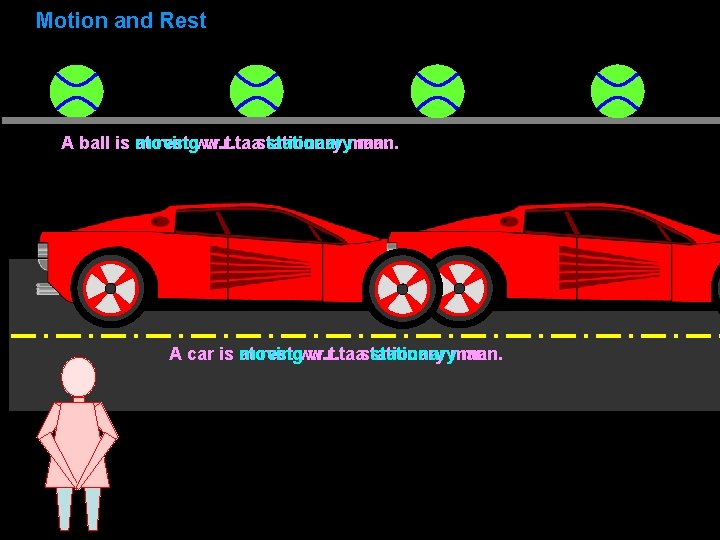Motion and Rest A ball is at moving rest w. r. t. aastationaryman. A car is at rest w. r. t. moving w. r. t. aastationaryman.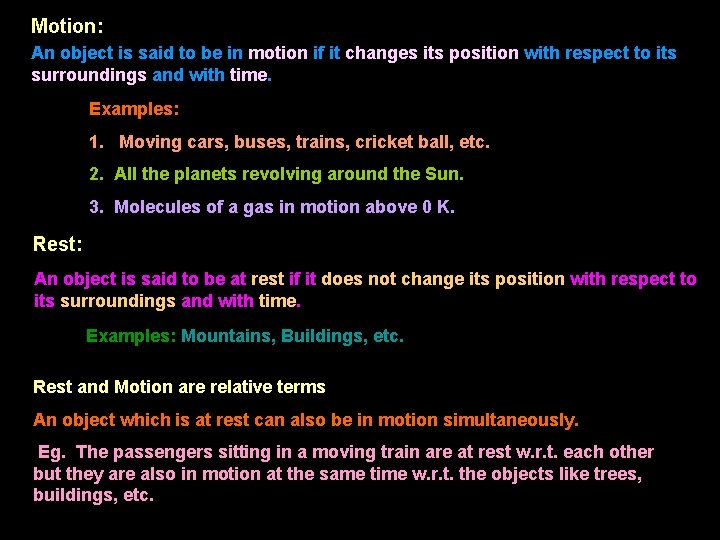Motion: An object is said to be in motion if it changes its position with respect to its surroundings and with time. Examples: 1. Moving cars, buses, trains, cricket ball, etc. 2. All the planets revolving around the Sun. 3. Molecules of a gas in motion above 0 K. Rest: An object is said to be at rest if it does not change its position with respect to its surroundings and with time. Examples: Mountains, Buildings, etc. Rest and Motion are relative terms An object which is at rest can also be in motion simultaneously. Eg. The passengers sitting in a moving train are at rest w. r. t. each other but they are also in motion at the same time w. r. t. the objects like trees, buildings, etc.Motion and Rest are Relative Terms Car is moving w. r. t. stationary man.Motion and Rest are Relative Terms Both the cars are at rest w. r. t. stationary man. Both the cars are moving w. r. t. a stationary man. Both the cars are at rest w. r. t. each other.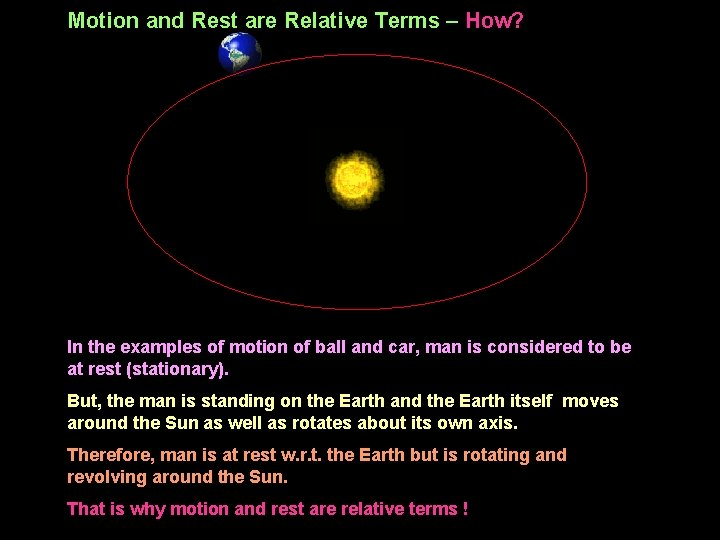Motion and Rest are Relative Terms – How? In the examples of motion of ball and car, man is considered to be at rest (stationary). But, the man is standing on the Earth and the Earth itself moves around the Sun as well as rotates about its own axis. Therefore, man is at rest w. r. t. the Earth but is rotating and revolving around the Sun. That is why motion and rest are relative terms !A ship is sailing in the ocean. Man-A in the ship is running on the board in the direction opposite to the direction of motion of the ship. Man-B in the ship is standing and watching the Man-A. Analyse the following cases to understand motion and rest ! 1. Man-A w. r. t. Man-B 2. Man-A w. r. t. ship 3. Man-B w. r. t. ship 4. Ship w. r. t. still water 5. Man-A w. r. t. still water 6. Man-B w. r. t. still water 7. Ocean w. r. t. the Earth 8. Ocean w. r. t. the Sun 9. Earth w. r. t. the Sun 10. Ship w. r. t. the Sun 11. The Sun w. r. t. Milky Way Galaxy 12. Milky Way Galaxy w. r. t. other galaxies Your imagination should not ever stop !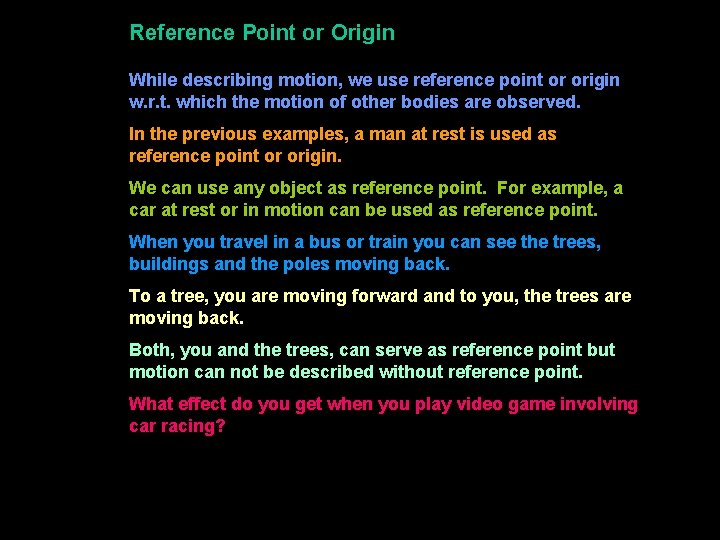Reference Point or Origin While describing motion, we use reference point or origin w. r. t. which the motion of other bodies are observed. In the previous examples, a man at rest is used as reference point or origin. We can use any object as reference point. For example, a car at rest or in motion can be used as reference point. When you travel in a bus or train you can see the trees, buildings and the poles moving back. To a tree, you are moving forward and to you, the trees are moving back. Both, you and the trees, can serve as reference point but motion can not be described without reference point. What effect do you get when you play video game involving car racing?Motion in a straight line The motion of a body may take place in one dimension, i. e. in a straight line. This can be represented graphically by plotting a graph between the position of the body and the time taken by it. This is called position-time graph. Origin, unit and direction of position measurement of an object (in km) -x -60 -50 -40 -30 -20 -10 0 10 20 30 40 50 60 70 +x 1. The distance measured to the right of the origin of the position axis is taken positive and the distance measured to the left of the origin is taken negative. 2. The origin for position can be shifted to any point on the position axis. 3. The distance between two points on position-axis is not affected due to the shift in the origin of position-axis.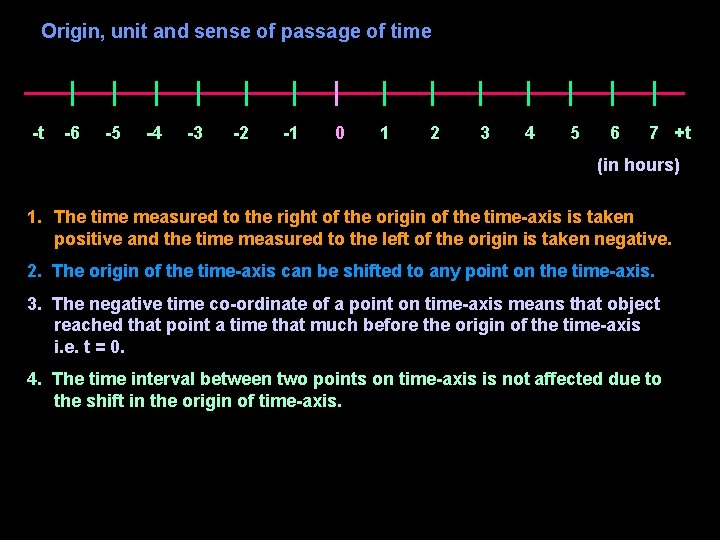Origin, unit and sense of passage of time -t -6 -5 -4 -3 -2 -1 0 1 2 3 4 5 6 7 +t (in hours) 1. The time measured to the right of the origin of the time-axis is taken positive and the time measured to the left of the origin is taken negative. 2. The origin of the time-axis can be shifted to any point on the time-axis. 3. The negative time co-ordinate of a point on time-axis means that object reached that point a time that much before the origin of the time-axis i. e. t = 0. 4. The time interval between two points on time-axis is not affected due to the shift in the origin of time-axis.When the same point is chosen as origins for position and time x = 0 km x = 30 km x = 40 km x = 55 km t =0 h t =6 h t=8 h t = 11 h A B C O Origin for position and time When the different points are chosen as origins for position and time x = -40 km x = -10 km x = 15 km t = -6 h t =0 h t=5 h O A Origin for time t=2 h B C Origin for position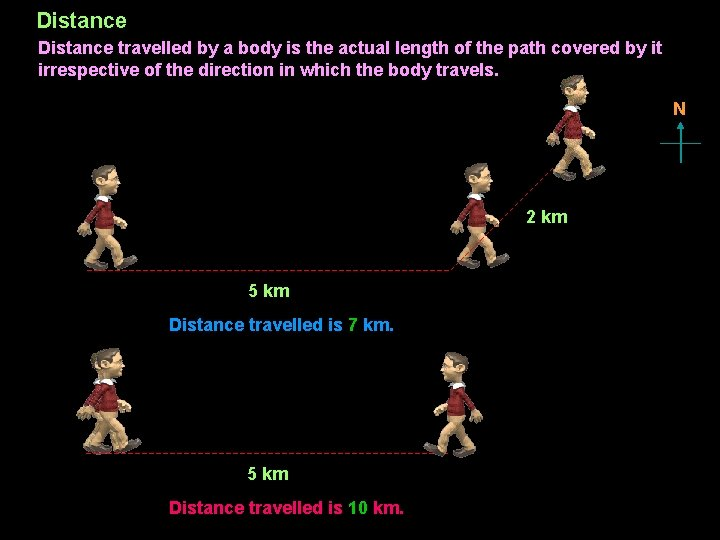Distance travelled by a body is the actual length of the path covered by it irrespective of the direction in which the body travels. N 2 km 5 km Distance travelled is 7 km. 5 km Distance travelled is 10 km.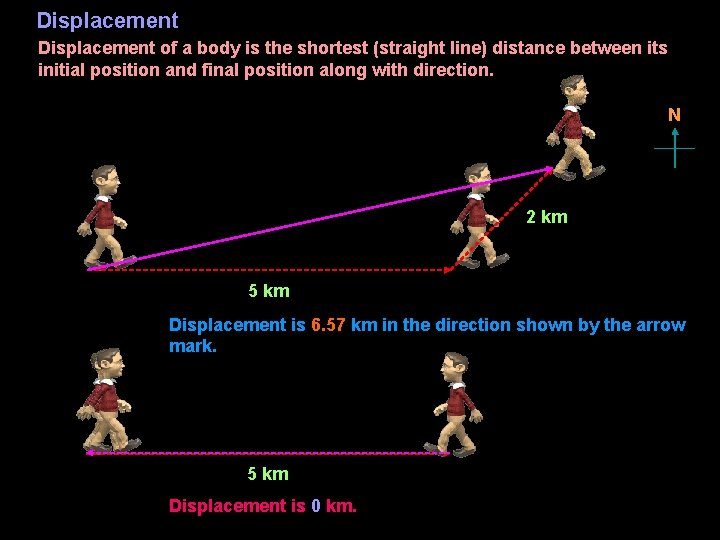Displacement of a body is the shortest (straight line) distance between its initial position and final position along with direction. N 2 km 5 km Displacement is 6. 57 km in the direction shown by the arrow mark. 5 km Displacement is 0 km.Distance A C Displacem ent B Conclusions about displacement: 1. The displacement is a vector quantity. 2. The displacement has units of length. 3. The displacement of an object in a given time interval can be positive, zero or negative. 4. The actual distance travelled by an object in a given time interval can be equal to or greater than the magnitude of the displacement. 5. The displacement of an object between two points does not tell exactly how the object actually moved between those points. 6. The displacement of a particle between two points is a unique path, which can take the particle from its initial to final position. 7. The displacement of an object is not affected due to the shift in the origin of the position-axis.S. No. Distance Displacement 1 Distance is a scalar quantity. Displacement is a vector quantity. 2 Distance travelled by a moving Final displacement of a moving body cannot be zero. body can be zero. Scalar quantity is a physical quantity which has magnitude only. Eg. : Length, Mass, Time, Speed, Energy, etc. Vector quantity is a physical quantity which has both magnitude as well as direction. Eg. : Displacement, Velocity, Acceleration, Momentum, Force, etc. Physical Quantity Physical quantity is a quantity which can be measured and expressed in magnitude (value with or without unit). Eg. : 1. Length can be measured and expressed as 5 m. 2. Relative Density can be measured and expressed as 0. 8Speed is defined as the time rate of change of distance of a body. or Speed is defined as the distance travelled by a body in unit time. Speed = Distance travelled Time taken If a body travels a distance ‘s’ in time ‘t’, then its speed ‘v’ is given by: Note: v= s t 1. Speed is a scalar quantity. 2. Speed is either positive or zero but never negative. 3. Speed of a running car is measured by ‘speedometer’. 4. Speed is measured in i) cm/s (cm s-1) in cgs system of units ii) m/s (m s-1) in SI system of units and iii) km/h (km. p. h. , km h-1) in practical life when distance and time involved are large.Uniform Speed A body has a uniform speed if it travels equal distances in equal intervals of time, no matter how small these time intervals may be. Uniform Motion A body has a uniform motion if it travels equal distances in equal intervals of time, no matter how small these time intervals may be. Variable Speed A body is said to be moving with variable speed, if it covers unequal distances in equal intervals of time, howsoever small these intervals may be. Non-uniform Motion A body has a non-uniform motion if it travels unequal distances in equal intervals of time.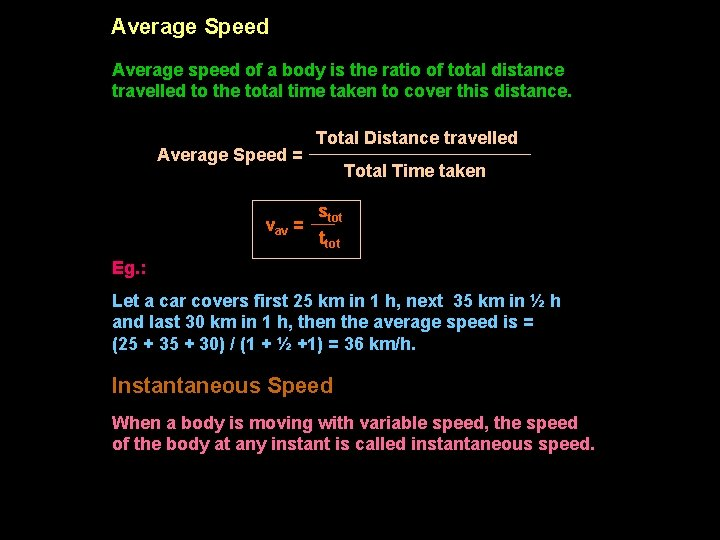Average Speed Average speed of a body is the ratio of total distance travelled to the total time taken to cover this distance. Average Speed = vav = Total Distance travelled Total Time taken stot ttot Eg. : Let a car covers first 25 km in 1 h, next 35 km in ½ h and last 30 km in 1 h, then the average speed is = (25 + 30) / (1 + ½ +1) = 36 km/h. Instantaneous Speed When a body is moving with variable speed, the speed of the body at any instant is called instantaneous speed.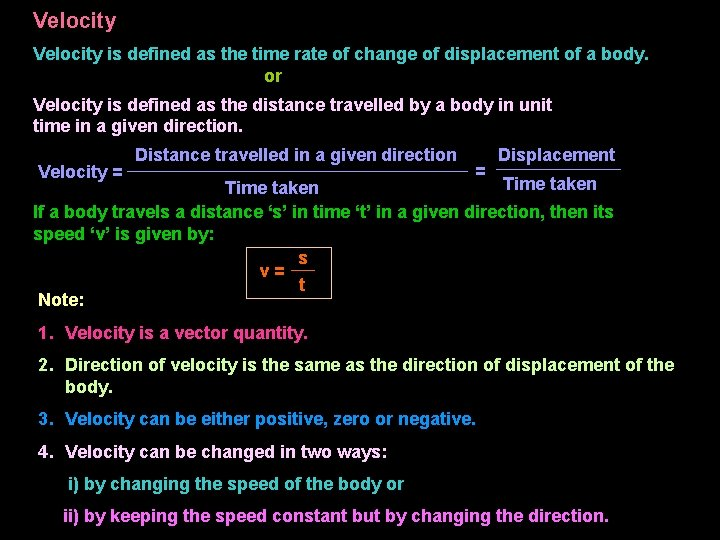Velocity is defined as the time rate of change of displacement of a body. or Velocity is defined as the distance travelled by a body in unit time in a given direction. Velocity = Distance travelled in a given direction = Displacement Time taken If a body travels a distance ‘s’ in time ‘t’ in a given direction, then its speed ‘v’ is given by: s v= t Note: 1. Velocity is a vector quantity. 2. Direction of velocity is the same as the direction of displacement of the body. 3. Velocity can be either positive, zero or negative. 4. Velocity can be changed in two ways: i) by changing the speed of the body or ii) by keeping the speed constant but by changing the direction.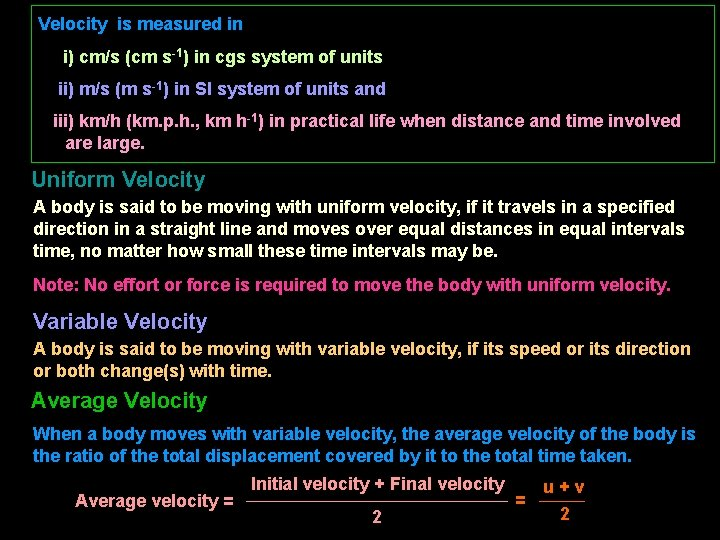Velocity is measured in i) cm/s (cm s-1) in cgs system of units ii) m/s (m s-1) in SI system of units and iii) km/h (km. p. h. , km h-1) in practical life when distance and time involved are large. Uniform Velocity A body is said to be moving with uniform velocity, if it travels in a specified direction in a straight line and moves over equal distances in equal intervals time, no matter how small these time intervals may be. Note: No effort or force is required to move the body with uniform velocity. Variable Velocity A body is said to be moving with variable velocity, if its speed or its direction or both change(s) with time. Average Velocity When a body moves with variable velocity, the average velocity of the body is the ratio of the total displacement covered by it to the total time taken. Average velocity = Initial velocity + Final velocity 2 = u+v 2Instantaneous Velocity When a body is moving with variable velocity, the velocity of the body at any instant is called instantaneous velocity. Difference between Speed and Velocity Speed 1. Speed is the time rate of change of distance of a body. Velocity 1. Velocity is the time rate of change of displacement of a body. 2. Speed tells nothing about the direction of motion of the body. 2. Velocity tells the direction of motion of the body. 3. Speed is a scalar quantity. 3. Velocity is a vector quantity. 4. Speed of the body can be positive or zero. 4. Velocity of the body can be positive, zero or negative. 5. Average speed of amoving body can never be zero. 5. Average velocity of a moving body can be zero.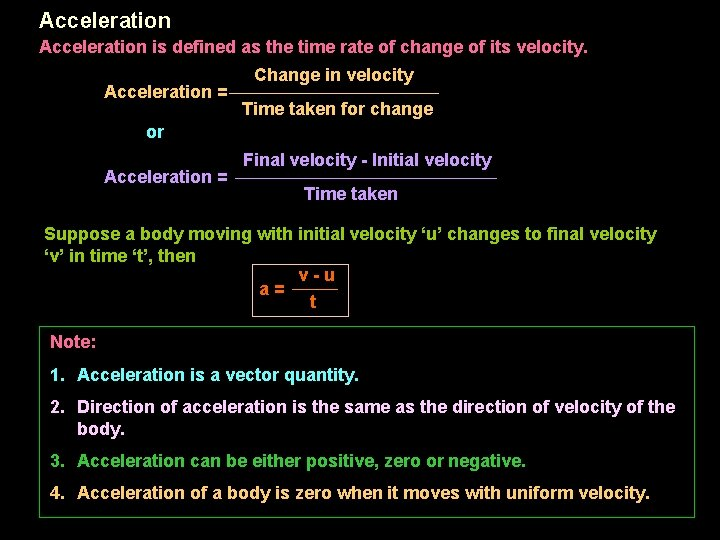Acceleration is defined as the time rate of change of its velocity. Acceleration = Change in velocity Time taken for change or Acceleration = Final velocity - Initial velocity Time taken Suppose a body moving with initial velocity ‘u’ changes to final velocity ‘v’ in time ‘t’, then v-u a= t Note: 1. Acceleration is a vector quantity. 2. Direction of acceleration is the same as the direction of velocity of the body. 3. Acceleration can be either positive, zero or negative. 4. Acceleration of a body is zero when it moves with uniform velocity.Acceleration is measured in i) cm/s 2 (cm s-2) in cgs system of units ii) m/s 2 (m s-2) in SI system of units and iii) km/h 2 (km h-2) in practical life when distance and time involved are large. Uniform Acceleration A body is said to be moving with uniform acceleration, if it travels in a straight line and its velocity increases by equal amounts in equal intervals of time. or A body has uniform acceleration if its velocity changes at a uniform rate.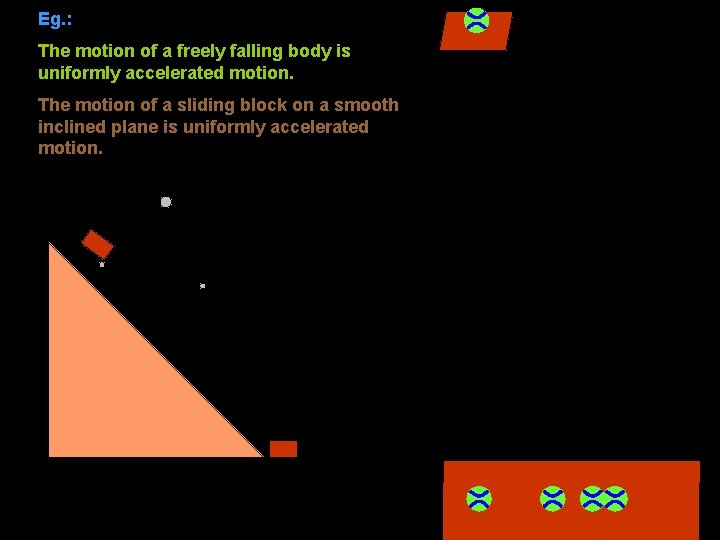Eg. : The motion of a freely falling body is uniformly accelerated motion. The motion of a sliding block on a smooth inclined plane is uniformly accelerated motion.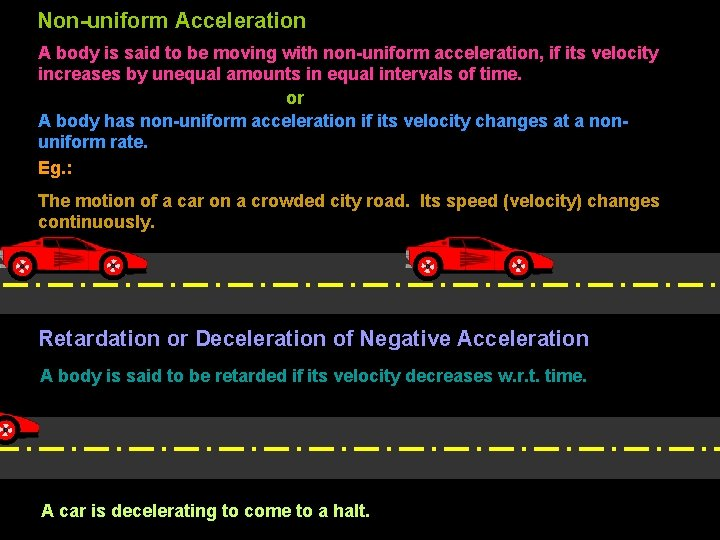Non-uniform Acceleration A body is said to be moving with non-uniform acceleration, if its velocity increases by unequal amounts in equal intervals of time. or A body has non-uniform acceleration if its velocity changes at a nonuniform rate. Eg. : The motion of a car on a crowded city road. Its speed (velocity) changes continuously. Retardation or Deceleration of Negative Acceleration A body is said to be retarded if its velocity decreases w. r. t. time. A car is decelerating to come to a halt.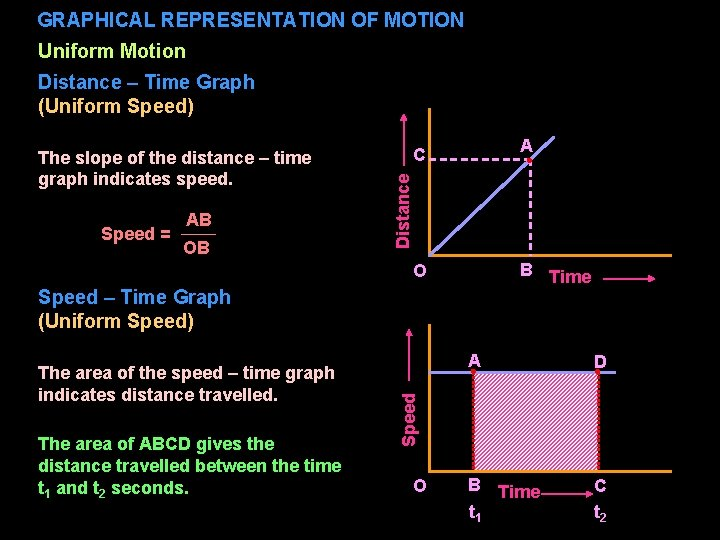GRAPHICAL REPRESENTATION OF MOTION Uniform Motion Distance – Time Graph (Uniform Speed) Speed = AB OB A C Distance The slope of the distance – time graph indicates speed. B Time O Speed – Time Graph (Uniform Speed) The area of ABCD gives the distance travelled between the time t 1 and t 2 seconds. A D B Time t 1 C t 2 Speed The area of the speed – time graph indicates distance travelled. ONon-Uniform Motion Non-uniform speed Distance – Time Graph (Uniform Acceleration) O Distance – Time Graph (Uniform Retardation) Time Distance Non-uniform speed O TimeSpeed (Velocity) – Time Graph (Uniform Acceleration) Acceleration = AB OB The area of AOB gives the distance travelled. Velocity The slope of the velocity – time graph indicates acceleration. A O B Time Speed (Velocity) – Time Graph: (Uniform Retardation) The slope of the velocity – time graph indicates retardation. Retardation = AO OB The area of AOB gives the distance travelled. Velocity A O B Time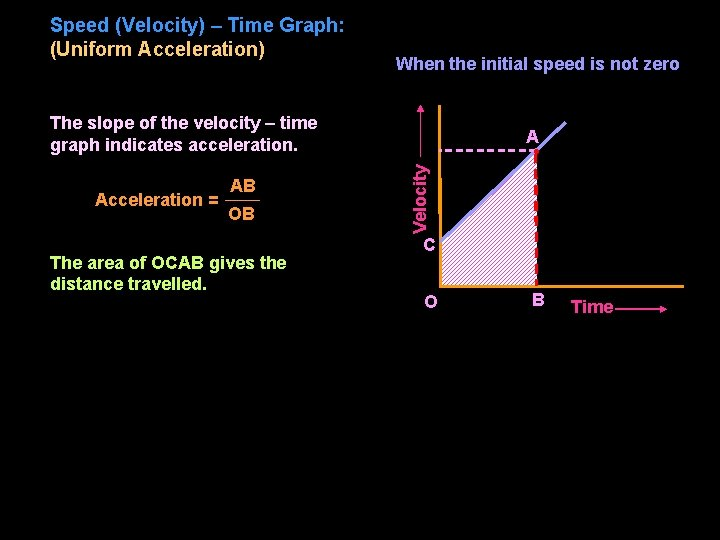Speed (Velocity) – Time Graph: (Uniform Acceleration) When the initial speed is not zero The slope of the velocity – time graph indicates acceleration. OB The area of OCAB gives the distance travelled. Velocity Acceleration = AB A C O B TimeEQUATIONS OF UNIFORMLY ACCELERATED MOTION u a s t Consider a body moving with initial velocity ‘u’ accelerates at uniform rate ‘a’. Let ‘v’ be the final velocity after time ‘t’ and ‘s’ be the displacement. First equation of motion We know that: Acceleration = Final velocity - Initial velocity Time taken a= Cross multiplying, or v-u t v – u = at v = u + at The equation v = u + at is known as the first equation of motion. v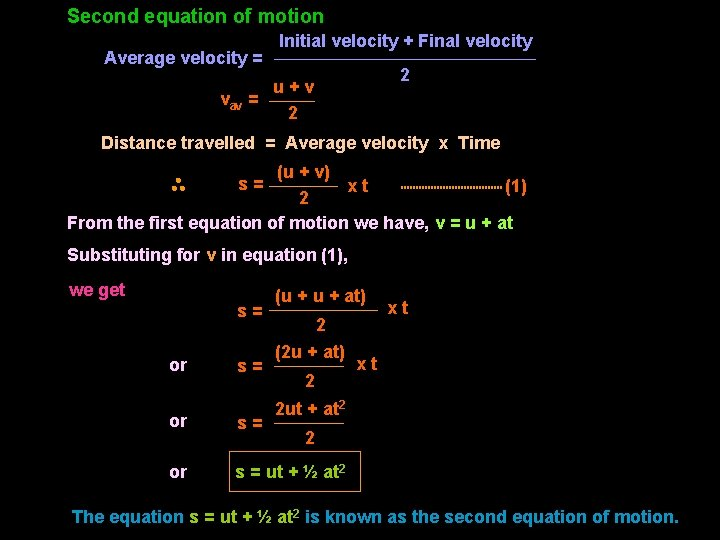Second equation of motion Average velocity = Initial velocity + Final velocity 2 u+v vav = 2 Distance travelled = Average velocity x Time (u + v) xt (1) 2 From the first equation of motion we have, v = u + at s= Substituting for v in equation (1), we get s= or s= (u + at) 2 (2 u + at) 2 xt xt 2 ut + at 2 or s= or s = ut + ½ at 2 2 The equation s = ut + ½ at 2 is known as the second equation of motion.Third equation of motion From the first equation of motion we have, v - u = at We know that: vav = (1) u+v 2 or u + v = 2 vav or v + u = 2 vav (2) Multiplying eqns. (1) and (2), we get v 2 - u 2 = 2 atvav or v 2 - u 2 = 2 as or v 2 = u 2 + 2 as vav x t = s The equation v 2 = u 2 + 2 as is known as the third equation of motion.EQUATIONS OF UNIFORMLY ACCELERATED MOTION BY GRAPHICAL METHOD First equation of motion Time taken for change a= a= a= BD AD AE OC OE - OA OC v-u a= t v – u = at or v = u + at Velocity (m/s) Acceleration = Change in velocity B v E u A O D t C Time (s)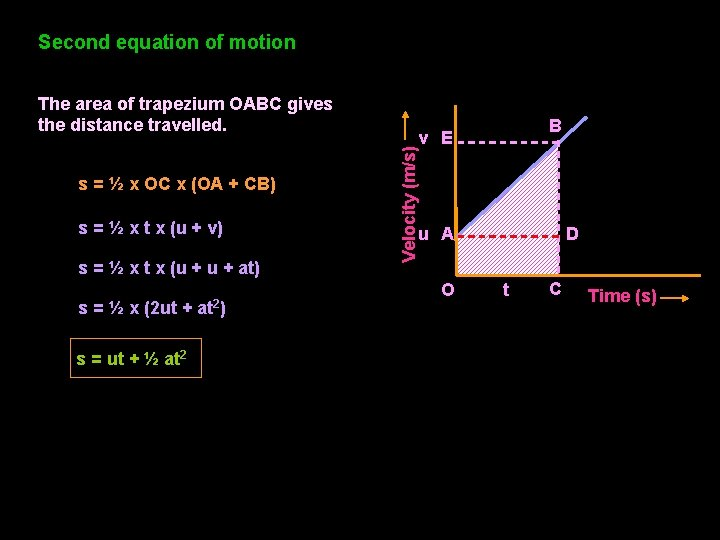Second equation of motion s = ½ x OC x (OA + CB) s = ½ x t x (u + v) s = ½ x t x (u + at) s = ½ x (2 ut + at 2) s = ut + ½ at 2 B v E Velocity (m/s) The area of trapezium OABC gives the distance travelled. u A O D t C Time (s)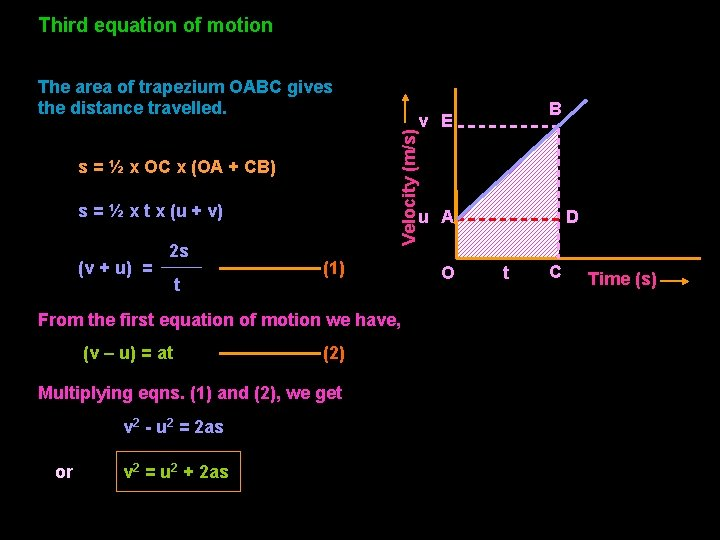Third equation of motion s = ½ x OC x (OA + CB) s = ½ x t x (u + v) (v + u) = 2 s t u A (1) From the first equation of motion we have, (v – u) = at (2) Multiplying eqns. (1) and (2), we get v 2 - u 2 = 2 as or v 2 = u 2 + 2 as B v E Velocity (m/s) The area of trapezium OABC gives the distance travelled. O D t C Time (s)UNIFORM CIRCULAR MOTION O The motion of a body in a circular or angular or curved path is called circular or angular or curvilinear motion. When a body moves in a circular path with uniform speed, its motion is called uniform circular motion. Note that the velocity changes at each and every instant and hence the body experiences acceleration. 2πr Velocity of a body in circular path = t r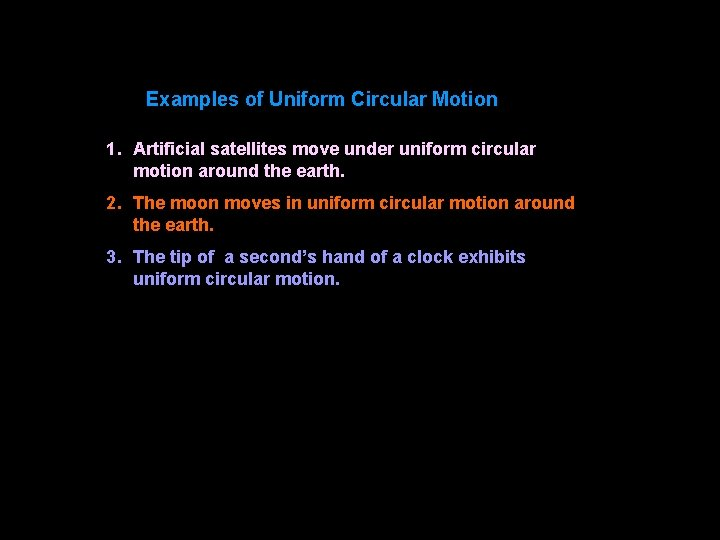Examples of Uniform Circular Motion 1. Artificial satellites move under uniform circular motion around the earth. 2. The moon moves in uniform circular motion around the earth. 3. The tip of a second’s hand of a clock exhibits uniform circular motion.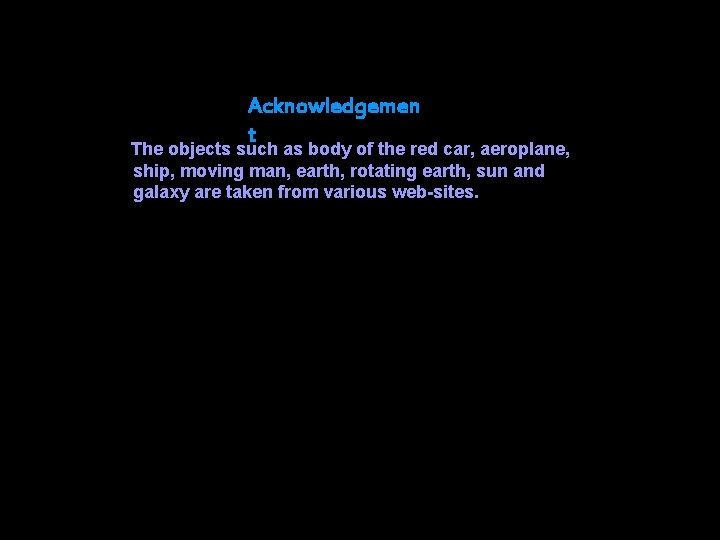Acknowledgemen t The objects such as body of the red car, aeroplane, ship, moving man, earth, rotating earth, sun and galaxy are taken from various web-sites.